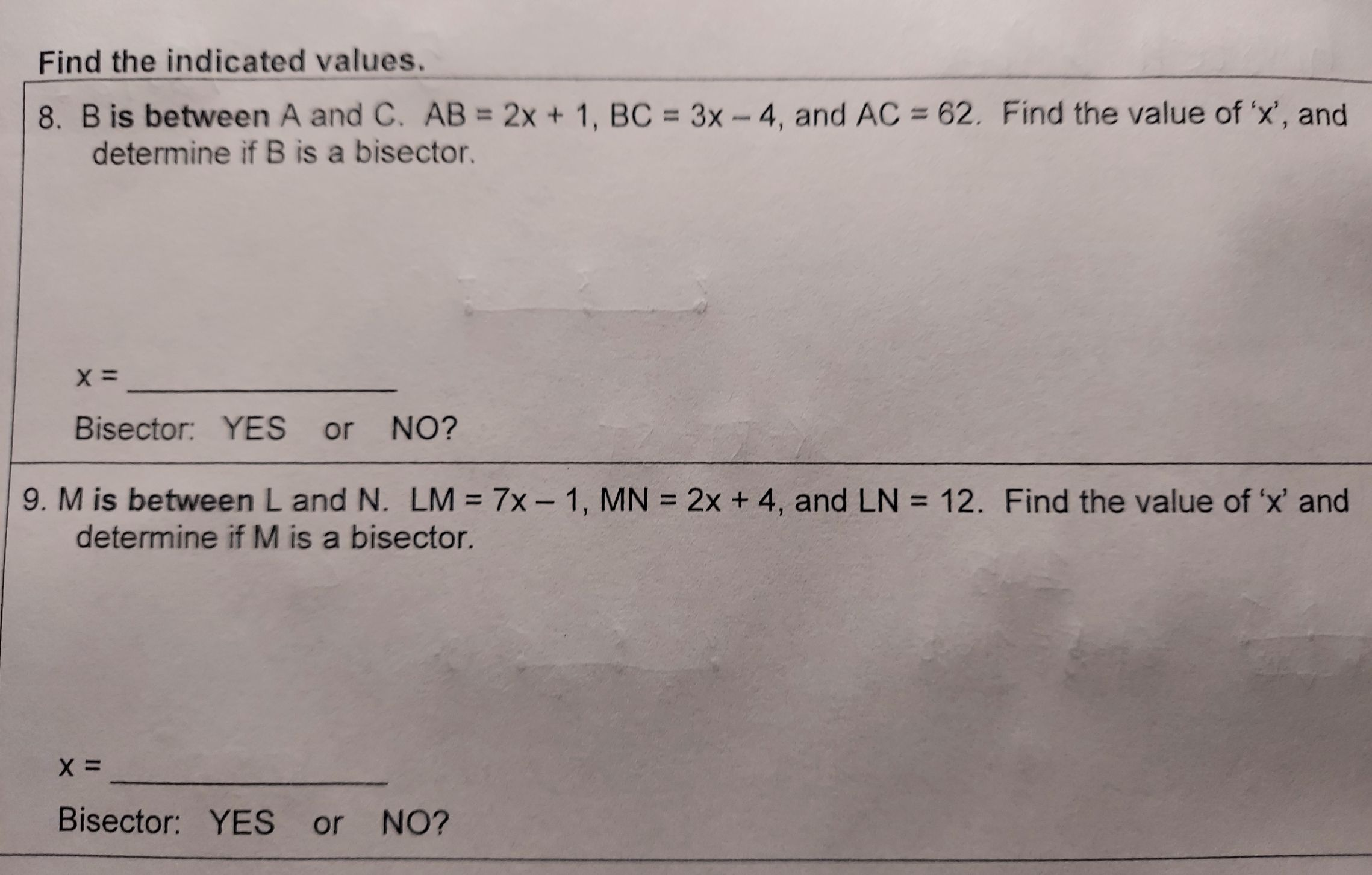### ¿Todavía tienes preguntas de matemáticas?

Pregunte a nuestros tutores expertos
Algebra
Pregunta8. $$B$$ is between $$A$$ and $$C . A B = 2 x + 1 , B C = 3 x - 4$$ , and $$A C = 62$$ . Find the value of ' $$x$$ ', and determine if $$B$$ is a bisector.

$$x =$$ Bisector: YES or NO?

9. $$M$$ is between $$L$$ and $$N . L M = 7 x - 1 , M N = 2 x + 4 ,$$ and $$L N = 12$$ . Find the value of ' $$x$$ ' and determine if $$M$$ is a bisector.

$$x =$$ Bisector: YES or NO?